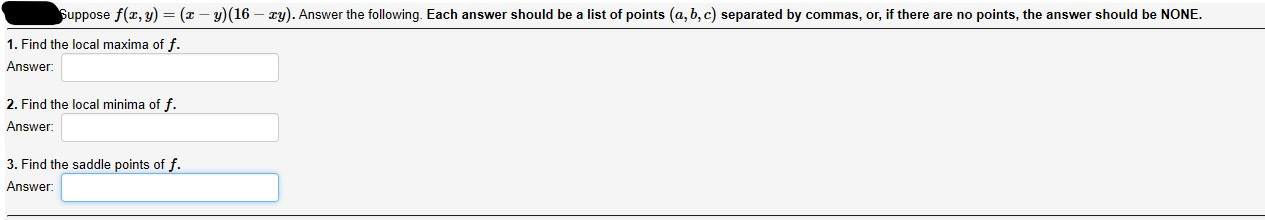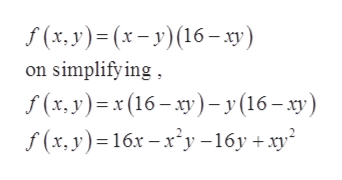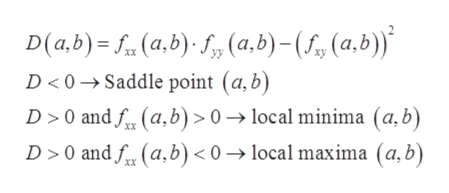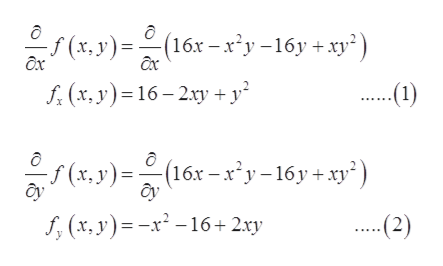# Suppose f(x, y) = (x – y)(16 – ry). Answer the following. Each answer should be a list of points (a, b, c) separated by commas, or, if there are no points, the answer should be NONE.1. Find the local maxima of f.Answer:2. Find the local minima of f.Answer:3. Find the saddle points of f.Answer:

Question
13 viewshelp_outlineImage TranscriptioncloseSuppose f(x, y) = (x – y)(16 – ry). Answer the following. Each answer should be a list of points (a, b, c) separated by commas, or, if there are no points, the answer should be NONE. 1. Find the local maxima of f. Answer: 2. Find the local minima of f. Answer: 3. Find the saddle points of f. Answer: fullscreen
check_circle

Step 1

Given, two variable functionhelp_outlineImage TranscriptioncloseS (x, y)= (x- y)(16– xy) on simplify ing , f (x, y)=x (16 – xy)-y(16– xy) f (x, y)=16x – x*y -16y + xy fullscreen
Step 2

In order to find local minima, local maxima and saddle point, differentiate partial and find the critical points and differentiate twice to classify.help_outlineImage TranscriptioncloseD(a,b) = f. (a,b)· f,» (a,b)- (f, (a,b))* D < 0 → Saddle point (a,b) D > 0 and f. (a,b) > 0 → local minima (a, b) D > 0 and f. (a,b) < 0 → local maxima (a, b) xX fullscreen
Step 3

Now, partial diffe...help_outlineImage Transcriptionclose(x,y) = (16x – x'y-16y + xy*) (16x – x²y –16y + xy²) * (x,y)= Ôx f. (x, y)=16 – 2xy + y² ..(1) (x,y)=(16x –x'y – 16y + xy*) ây f, (x, y)= -x² - 16+2.xy -(2) fullscreen

### Want to see the full answer?

See Solution

#### Want to see this answer and more?

Solutions are written by subject experts who are available 24/7. Questions are typically answered within 1 hour.*

See Solution
*Response times may vary by subject and question.
Tagged in

### Derivative